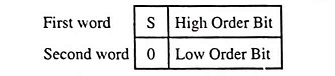## What is Double Precision System?

For any computer, the word length is fixed. Most present day computers are 16 bit and in a 16 bit computer, that is, in a computer with 16-bit word length, only numbers from +216-1 – 1 (i.e., +32.767) to -216-1 (i.e., -32,768) can be stored in each register. To store numbers larger than these numbers, two storage locations need to be used, that is, each such number has to be stored in two registers. This is known as double precision system. Leaving the MSB which is the sign bit, this allows a 31-bit number length with two 16-bit registers. The format isS is the sign bit and 0 is a zero.

If still larger numbers are to be stored, three registers are used to store each number. This is known as triple precision system.

Scroll to Top